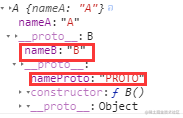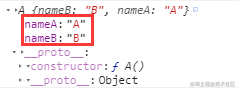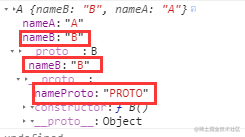# 小议JS原型链、继承

### 原型链

``````var A = function(){
this.name="xiaoming";
}
A.prototype.age=9;
var a = new A();
console.log(a.age); //9

``````a.__proto__ === A.prototype; //true
A.prototype.constructor===A; //true
A.prototype.__proto__===Object.prototype; //true
Object.prototype.__proto__===null; //true

``````var A = function(){
this.name="xiaoming";
}
var a = new A();
A.prototype.age=9;
var b = new A();
console.log(a.age); //9
console.log(b.age); //9

a、b都可以访问A原型链上的属性age。

Function和Object比较特殊，他们既是对象又是函数，两者内部同时含有proto和prototype属性，可看下面代码：

``````Object.__proto__ === Function.prototype //true
Object.__proto__ === Function.__proto__//true
Object.prototype === Function.prototype.__proto__ // true
Function instanceof Object //true
Object instanceof Function //true

### 原型链继承

`A.prototype=new B()`；关于new可以看下我另一篇文章this那些事

``````function B(){
this.nameB='B';
}
B.prototype.nameProto="PROTO";
function A(){
this.nameA="A";
}
A.prototype=new B(); //原型链继承：A继承B
var a=new A();
console.log(a);上段代码A继承B，`通过A构造函数new的示例a不仅可以继承B（可访问nameB），而且可以继承B原型上的属性（nameProto），且是所有实例共享的。`

### 构造继承

``````function B(){
this.nameB='B';
}
B.prototype.nameProto="PROTO";
function A(){
B.call(this); //A继承B，只举了call的例子，apply、bind类似
this.nameA="A";
}
var a=new A();
console.log(a);根据a的打印结果，我们看到nameB和nameA是同一层级，虽然实现了A继承B，但是通过a的结构看不出来，而且`无法继承B原型链上的属性nameProto`，不过它的好处是可以`多继承`，可以通过`C.call(this)`继承C。

### 组合继承

``````function B(){
this.nameB='B';
}
B.prototype.nameProto="PROTO";
function A(){
B.call(this); //构造继承
this.nameA="A";
}
A.prototype=new B(); //原型链继承：A继承B
var a=new A();
console.log(a);观察a的打印结果，似乎真的解决了上述两个问题，它也引入了一个新的问题：`nameB属性有两个`，这样造成了资源浪费（存储占用内存）。

### 原型式继承

``````function objectCreate(obj){
function F(){};
F.prototype = obj;
return new F();
}
var a=objectCreate(A.prototype);

1. 使用`__proto__``A.prototype.__proto__=B.prototype`
2. 使用`Object.create``A.prototype=Object.create(B.prototype)`